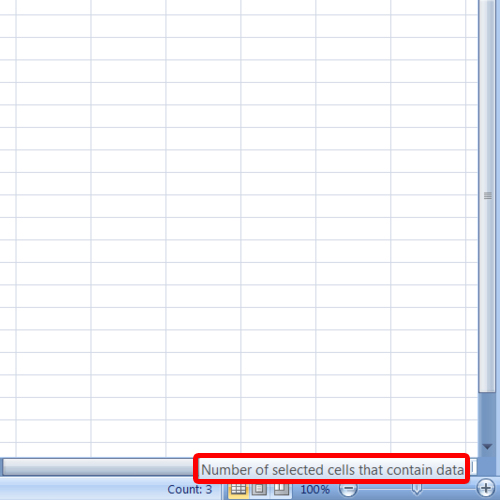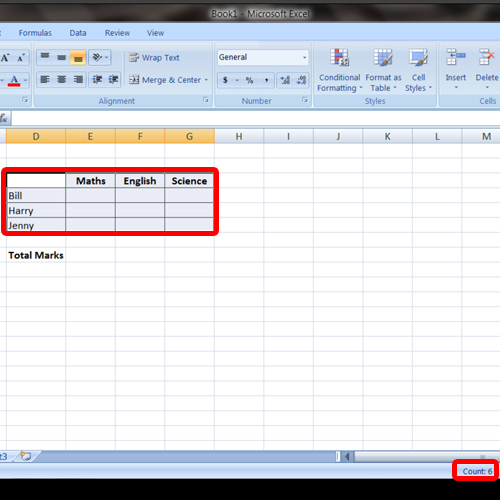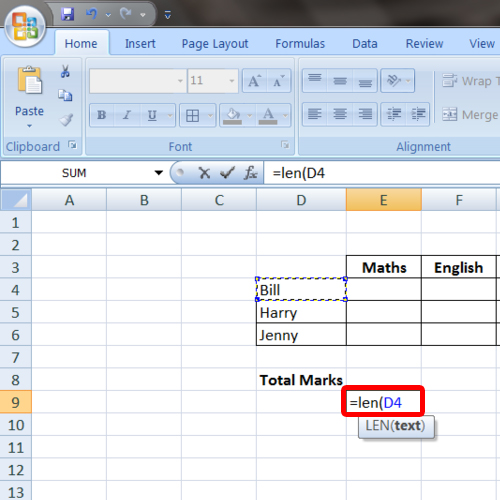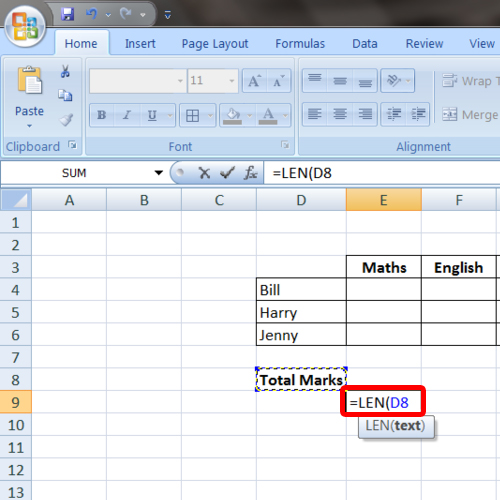## How to Count Cells in Excel

In this tutorial, we will teach you how to count cells in Excel. Excel doesn’t count the blank cells when counting. It only counts those cells which contain data in them.
Step 1 – Select cells for counting
Follow this step by step guide in order to learn how to count cells in excel. First of all, open an excel file that you can work with. For the purpose of this tutorial, we have prepared a sample data. We will select the three subjects on the top and the total count can be viewed at the bottom of the screen. If we hover over the count region, we will be displayed with the following text:
Number of selected cells that contain data.
In this way, we can count cells in excel which contain data.Step 2 – Select entire table
Next we will select the entire table. You will notice that the counter only counts those cells which contain data and ignores the blank cells.Step 3 – Use len function on one word
Now we will show you how to use the len function. Type in
“=lens( and then select the cell whose characters you want to count.
For the purpose of this tutorial, we will count the letters in the student names.Step 4 – Use len function on cell containing two words
However, if a cell contains two words or more, the len function will count the space between the words as a character. For example, if we apply the len function on “total marks”, it will give us a count of 11 not 10. In this manner, you can count cells in excel or the characters in a cell.